# How to Sort Data Automatically in Excel (New data is entered or data changed)

This post will guide you how to sort data by column values automatically with VBA Macro in Excel. How do I sort values automatically when a new value or data is entered in Excel 2013/2016. How to automatic sort data when data is updated in Excel.

## Automatically Sorting Values When Data Changes

Assuming that you have a list of data in range B1:B5, in which contain sale values. And you want to sort those values in your data. You can use Sort function to sort those values. But when you add additional data or change the current values in this range, the order in the range will not be changed automatically.

For example, you try to add a new data set into the range B1:B5, it won’t change the order automatically. If you want to sort this range with new data, you need to re-perform the sort function.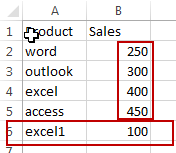So you may be think if there is a good way to automatically sort those values in Excel. Of course, the answer is yes. You can use a VBA Macro to achieve the result. Here are the steps:

#1 right click on the sheet tab, and select view code from the popup menu list. And the Microsoft Visual Basic for Applications window will appear.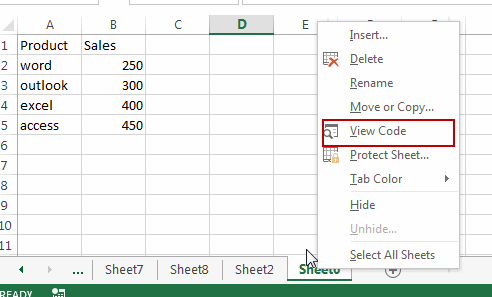#2 copy the following codes into the code window in VBA window. Save and close it.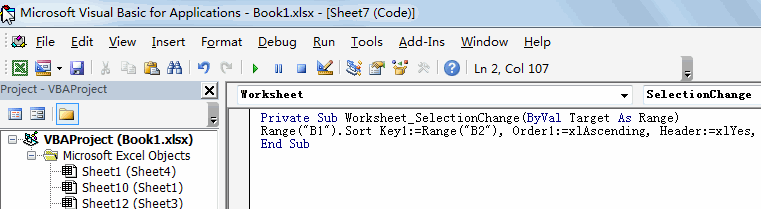```Private Sub Worksheet_SelectionChange(ByVal Target As Range)
Range("B1").Sort Key1:=Range("B2"), Order1:=xlAscending, Header:=xlYes, OrderCustom:=1, MatchCase:=False, Orientation:=xlTopToBottom
End Sub```

#3 you can go back to the original worksheet, and enter a new sale value in Cell B6 or you can also modify the original sale values. You should notice that the values will be sorted automatically.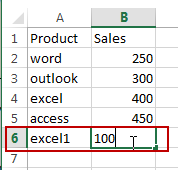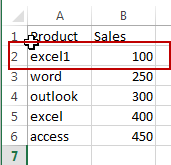The above VBA Macro code can be used to sort a single column with Header in Excel. And if you want to sort a single column without header, you can use the following codes:

```Private Sub Worksheet_SelectionChange(ByVal Target As Range)
End Sub```

Related Posts
VBA Macro For VLOOKUP From Another Sheet

In the previous post, you should know that how to fix or remove the #N/A error when using VLOOKUP formula to lookup value from another sheet. And this post will show you how to use VBA code to vlookup data ...

How To Insert Comments in Protected Worksheet in Excel

This post will show you how to allow comments in a protected worksheet in Excel. You can easily to insert comments into cells in a normal worksheet in Excel, but if want to insert a comment in a worksheet that ...

How To Convert Text to Upper Cases(Using VBA) in Excel

This post will show you how to switch from lower case to upper case in Excel. and I am going to show you two different ways of converting text to upper cases using formula or VBA macro in Excel 2013,Excel ...

How To Hide Every Other Row in Excel (Using VBA)

This post will show you how to hide alternate rows or columns in Excel or how to hide every third, fourth, fifth row or column in Excel. If you want to hide every other row in your current worksheet, how ...

How to Disable the Save As Prompt in Excel

This post will show you how to use a VBA Macro to save an Excel file and overwrite any existing file without a prompt so that you are going to get the little window that says file already exists do ...

How to Count Cells that Contain even or odd numbers in Excel

This post will guide you how to count the number of cells that contain odd or even numbers within a range of cells using a formula in Excel 2013/2016.How do I count cells that contain odd numbers through the use ...

How to Count Cells that Contain negative Numbers in Excel

This post will guide you how to count the number of cells that contain negative numbers within a range of cells using a formula in Excel 2013/2016.You can count the number of negative numbers in your data using easy functions ...

How to Count Cells Are Not Blank or Empty in Excel

This post will guide you how to count cells that are not blank or empty in a given range cells using a formula in Excel 2013/2016.How do I count the number of cells that are not blank in a particular ...

How to Count Cells Less Than a Specific Value in Excel

This post will guide you how to count the number of cells less than a particular numeric value in a given range cells using a formula in Excel 2013/2016. How do I count cells that are less than a specific ...

How to Count Cells Greater Than a Specific Value in Excel

This post will guide you how to count the number of cells greater than a particular numeric value in a given range cells using a formula in Excel 2013/2016. How do I count cells that are greater than a specific ...

Sidebar